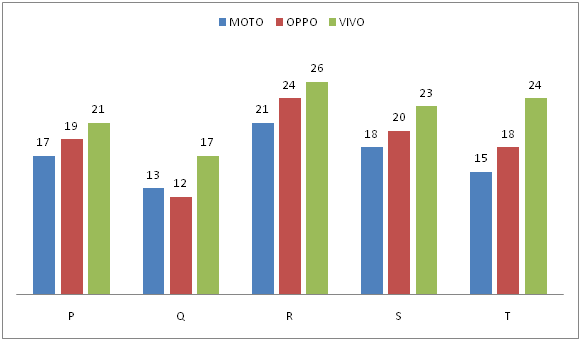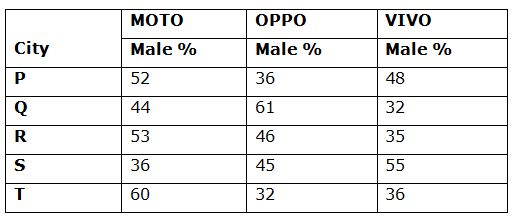# IBPS RRB Clerk/EPFO Assistant Prelims Quantitative Aptitude Questions 2019 (Day-09)

Dear Aspirants, Our IBPS Guide team is providing new series of Quantitative Aptitude Questions for IBPS RRB Clerk/EPFO Assistant Prelims 2019 so the aspirants can practice it on a daily basis. These questions are framed by our skilled experts after understanding your needs thoroughly. Aspirants can practice these new series questions daily to familiarize with the exact exam pattern and make your preparation effective.

##### EPFO Assistant Prelims Free Mock Test
[WpProQuiz 6705]

1) To do a certain work Livingston is 100/3% more efficient than Raju. They started to work alternatively on the same work starting from Livingston on first day and Raju on second day and finished 50% of the work in 40 days. Find the time taken by Livingston alone to complete the work.

a) 70 days

b) 46 days

c) 24 days

d) 35 days

e) 98 days

2) 7 typists working 8 hours a day can type a book in 20 days. How many days will 4 typists take to do the same job, each working 7 hours a day?

a) 53 days

b) 14 days

c) 40 days

d) 15 days

e) 20 days

3) If two dice are tossed, what is the probability that the total score is composite number?

a) 5/12

b) 9/12

c) 7/12

d) 3/12

e) 8/12

4) A person invests Rs 40000 in two different parts, one at 10% per annum simple interest and the other at 20% per annum compound interest. If at the end of two years he gets a total interest of Rs 8580, then what amount did he invest under compound interest (approximately)?

a) Rs 36983

b) Rs 37583

c) Rs 37932

d) Rs 37893

e) Rs 37937

5) Marked price of an article is Rs 2600 more than the cost price. When the same article is sold at a discount of Rs 1000 then the percentage profit earned is 20%. For earning a profit of 25, the article should be sold at what price?

a) Rs 2000

b) Rs 3000

c) Rs 5000

d) Rs 4000

e) Rs 3500

Directions (Q. 6 – 10) Study the following information carefully and answer the given questions:

Following bar graph shows the number of mobile users (in thousands) of three brands (i.e., MOTO, OPPO and VIVO) in different cities and the table shows the percentage of males using three brands of mobiles.6) Total number of female mobile users of MOTO and OPPO brands in the city P and T together is approximately what percentage of total number of male mobile users of OPPO and VIVO brands in the city Q and R together?

a) 117 %

b) 125 %

c) 103 %

d) 96 %

e) 88 %

7) Find the ratio between the total female mobile users of MOTO and VIVO brands of city Q and R together to that of total female mobile users of OPPO and VIVO brands of city S and T together?

a) 2897: 3245

b) 4375: 3667

c) 4561: 4895

d) 3243: 3896

e) None of these

8) Find the difference between the total male mobile users of all the given brands for city P, Q and R together to that of total female mobile users of all the given brands for city R, S and T together?

a) 39870

b) 30690

c) 34560

d) 30890

e) None of these

9) Total number of mobile users of city R and S together is approximately what percentage more/less than the total number of mobile users of city P, Q and T together for all the given brands?

a) 23 % more

b) 27 % less

c) 31 % more

d) 15 % less

e) 25 % more

10) Find the average male mobile users of OPPO brands in all the given cities together?

a) 8154

b) 6578

c) 7992

d) 9156

e) None of these

Direction (1-5) :

Ratio of efficiency of Livingston and Raju= 4:3

Then work done by Livingston= 4x units and work done by Raju=3x units

Since, 50% of the work completed in 40 days, 100% of the work will be completed in 80 days.

If they both work together, 7x units will be completed in two days.

So in 80 days, 80/2*7x units will be completed.

Hence the whole work will be completed by Livingston= (80*7x)/(2*4x)

= 70 days.

Let the number of days be x.

Then 7*8*20=4*7*x

x= 40 days

Possibility of getting number =

(1,3),(1,5),(2,2),(2,4),(2,6),(3,1),(3,3),(3,5),(3,6),(4,2),(4,4),(4,5),(4,6),(5,1),(5,3),(5,4),(5,5),(6,2),(6,3),(6,4),(6,6)

Thus probability of getting composite number=21/36=7/12

Let the amount invested by the person in the first part be Rs x.

Then, Remaining amount= 40000-x

According to the question,

[(x*10*2)/100] + (40000-x) [(1+(20/100)2-1)] = 8580

(x/5)+ (40000-x) (11/25) = 8580

After solving this we get x= Rs 37583

Given that Marked price- Cost price= Rs 2600

Then 2600= profit + discount

Thus profit= 2600-1000= 1600

(i.e.) 20% of the profit= Rs 1600

Then 25% of the profit= (1600/20)*25

= Rs 2000

Direction (6-10) :

Total number of female mobile users of MOTO and OPPO brands in the city P and T together

= > [17*(48/100) + 19*(64/100) + 15*(40/100) + 18*(68/100)] thousands

= > [8.16 + 12.16 + 6 + 12.24] thousands

= > 38560

Total number of male mobile users of OPPO and VIVO brands in the city Q and R together

= > [12*(61/100) + 17*(32/100) + 24*(46/100) + 26*(35/100)] thousands

= > [7.32 + 5.44 + 11.04 + 9.10] thousands

= > 32900

Required % = (38560/32900)*100 = 117.2 % = 117 %

The total female mobile users of MOTO and VIVO brands of city Q and R together

= > [13*(56/100) + 17*(68/100) + 21*(47/100) + 26*(65/100)] thousands

= > [7.28 + 11.56 + 9.87 + 16.90] thousands

= > 45610

The total female mobile users of OPPO and VIVO brands of city S and T together

= > [20*(55/100) + 23*(45/100) + 18*(68/100) + 24*(64/100)] thousands

= > [11 + 10.35 + 12.24 + 15.36] thousands

= > 48950

Required ratio = 45610: 48950 = 4561: 4895

The total male mobile users of all the given brands for city P, Q and R together

= > [17*(52/100) + 19*(36/100) + 21*(48/100) + 13*(44/100) + 12*(61/100) + 17*(32/100) + 21*(53/100) + 24*(46/100) + 26*(35/100)] thousands

= > [8.84 + 6.84 + 10.08 + 5.72 + 7.32 + 5.44 + 11.13 + 11.04 + 9.10] thousands

= > 75510

The total female mobile users of all the given brands for city R, S and T together

= > [21*(47/100) + 24*(54/100) + 26*(65/100) + 18*(64/100) + 20*(55/100) + 23*(45/100) + 15*(40/100) + 18*(68/100) + 24*(64/100)] thousands

= > [9.87 + 12.96 + 16.9+ 11.52 + 11 + 10.35 + 6 + 12.24 + 15.36] thousands

= > 106200

Required difference = 106200-75510 = 30690

Total number of mobile users of city R and S together for all the given brands

= > [21 + 24 + 26 + 18 + 20 + 23] thousands

= > 132000

Total number of mobile users of city P, Q and T together for all the given brands

= > [17 + 19 + 21 + 13 + 12 + 17 + 15 + 18 + 24] thousands

= > 156000

Required % = [(156000 – 132000)/156000]*100 = 15.38 % = 15 % less

Total male mobile users of OPPO brands in all the given cities together

= > [19*(36/100) + 12*(61/100) + 24*(46/100) + 20*(45/100) + 18*(32/100)] thousands

= > [6.84 + 7.32 + 11.04 + 9 + 5.76] thousands

= > 39960

Required average = 39960/5 = 7992